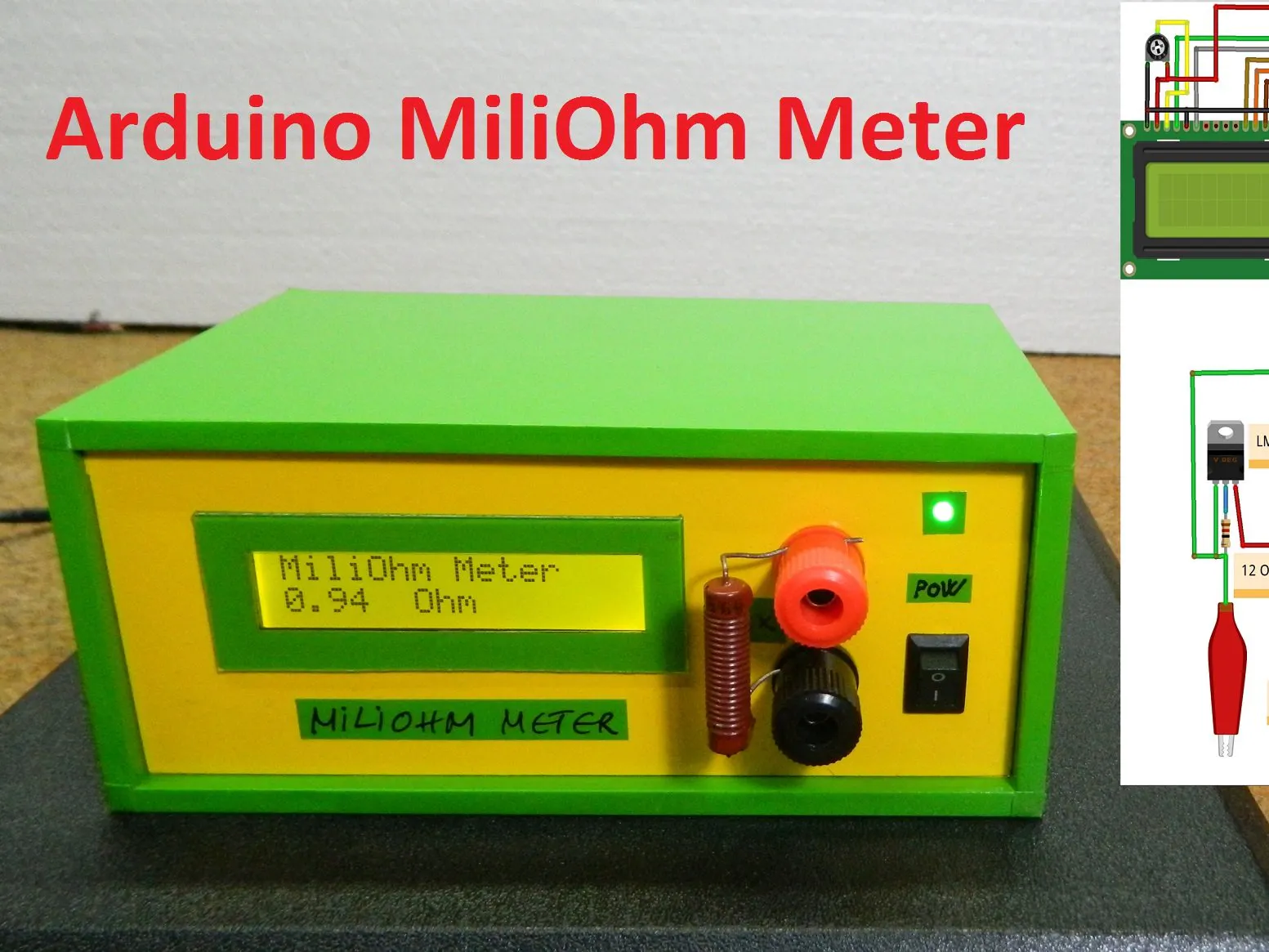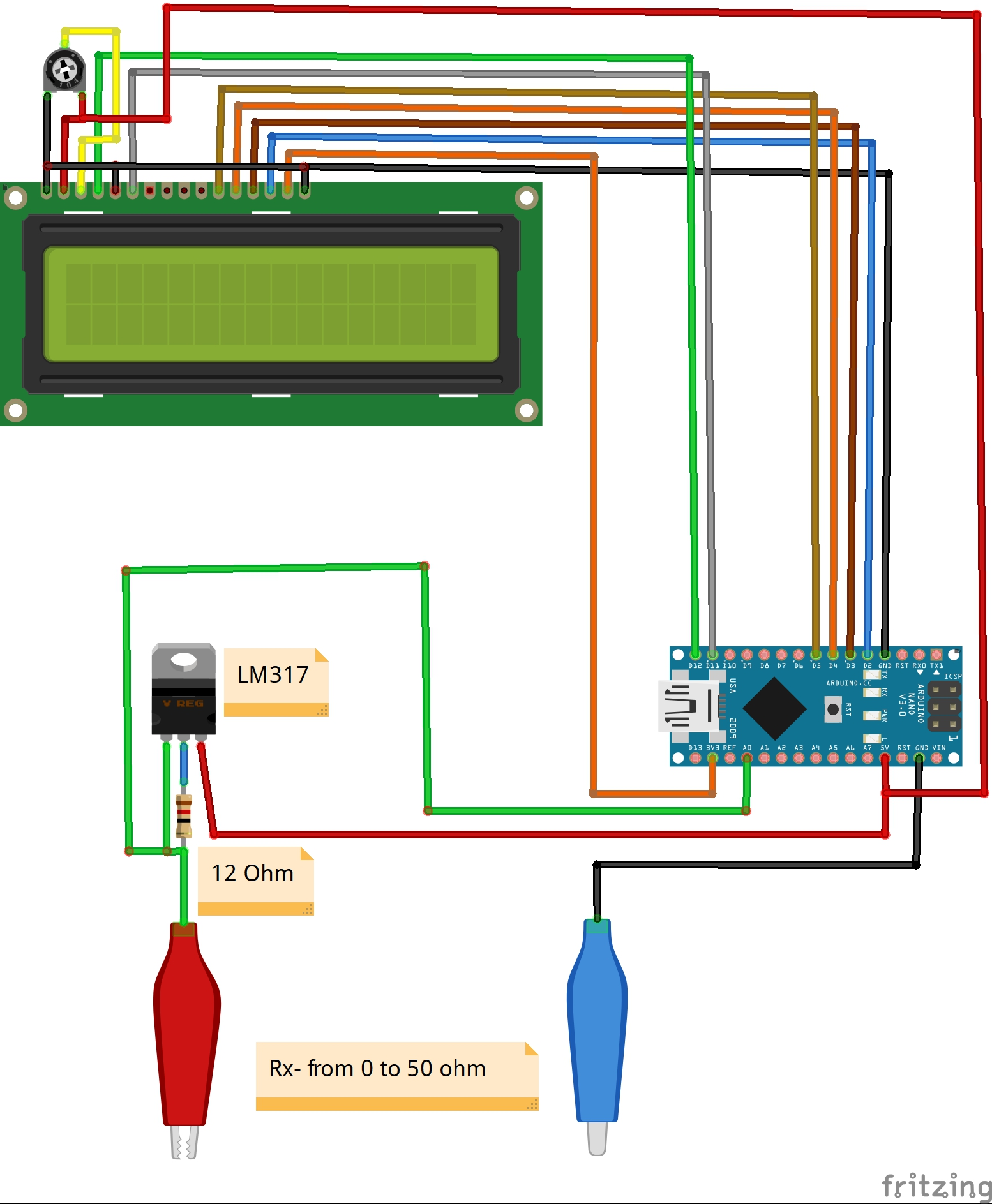Project tutorial# DIY Miliohmmeter for Measure Low Value Resistances © GPL3+

This device serves for accurate measurement of small resistances in the range of 0.1 ohm up to 50 ohms.

• 4,579 views
• 1 comment
• 11 respects

## Necessary tools and machinesSoldering iron (generic)

## Apps and online services

Measuring low resistances with a standard multimeter is difficult and very inaccurate. This device solves this problem and serves for accurate measurement of small resistances in the range of 0.1 ohm up to 50 ohms.

It is very simple and easy to make and contains the following components:

- Arduino Nano microcontroller

- 16x2 LCD display

- LM317 voltage regulator

- Potentiometer

- and resistor 12 Ohm

It consists of constant current source of 100mAmp. Constant current source is built using LM317:

Iconst = Vref / R where: Vref of LM317 is 1.25V

Iconst = 1.25 / R

Iconst = 104mAmp

Measuring voltage drop across a resistor having constant current gives us resistance value (R = V / I), Where:

I = 100mAmp as we are using constant current source of 100mAmp

V = Measured by Arduino

This code and circuit will not work for values more than 50 Ohm as 50 * 100mA = greater than 5V.

The accuracy of this Milliometer is satisfactory and mostly depends on the accuracy of the 12 ohm resistor and the stabilized voltage of 5 volts. Finally, the device is placed in a suitable box and is another useful tool in your laboratory.

## Code

##### CodeC/C++
```#include<LiquidCrystal.h>
LiquidCrystal lcd(12, 11, 5, 4, 3, 2);

void setup() {
// initialize serial communication at 9600 bits per second:
Serial.begin(9600);
lcd.begin(16, 2);
lcd.print("MiliOhm Meter");
}

// the loop routine runs over and over again forever:
void loop() {
// read the input on analog pin 0:

// Convert the analog reading (which goes from 0 - 1023) to a voltage (0 - 5V):
float voltage = sensorValue * (5.0 / 1024.0);

float Rx = voltage / 0.104;

if (Rx > 41) {

lcd.setCursor(0,1);
lcd.print( " Out of Range ");

delay(1000);
}
if (Rx < 40) {
lcd.setCursor(0,1);
lcd.print( Rx);

lcd.setCursor(5, 1);
lcd.print(" Ohm      ");
delay(1000);
}
}
```

## Schematics#### DIY Simple Frequency Meter Up to 6.5MHz

Project tutorial by Mirko Pavleski

• 14,074 views
• 19 respects

#### DIY Autorange Ohmmeter

Project tutorial by Mirko Pavleski

• 6,701 views
• 1 comment
• 13 respects

#### DIY Simple Autorange Capacitance Meter (10pF-10000microF)

Project tutorial by Mirko Pavleski

• 12,156 views
• 20 respects

#### DIY FFT Audio Spectrum Analyzer

Project tutorial by Mirko Pavleski

• 20,923 views
• 21 respects

#### DIY 6.5MHz Frequency Meter with MAX7219 7-Segment LED Module

Project tutorial by Mirko Pavleski

• 4,157 views
• 1 comment
• 9 respects

#### DIY 10Hz-50kHz Oscilloscope on 128x64 LCD Display

Project tutorial by Mirko Pavleski

• 16,549 views